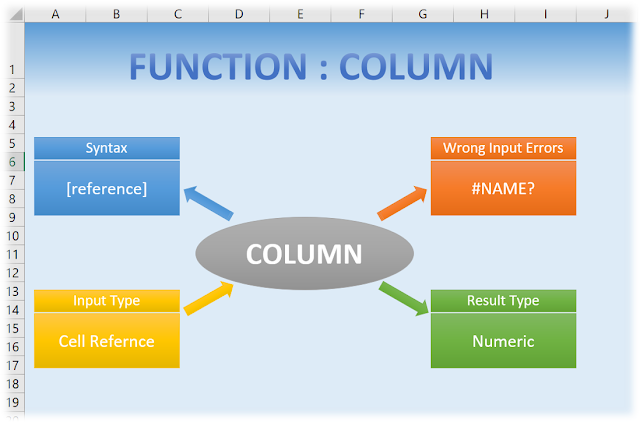# COLUMN

## “COLUMN”

Used for – “COLUMN” function in MS Excel is used to get the absolute position of the column of the reference cell.
Syntax – COLUMN([reference])

[reference] – This is an optional field as it is written in square brackets. It is a address of any cell for which we want to know the absolute position. The result of the this function is a numeric. If we do not put any reference it will consider the cell in which this function is typed and give the absolute position of the same.

Example – Following example will clear the COLUMN function:-

In below table formulas are shown which which reflects the various reference type for the function COLUMN:-

 =COLUMN(A1) =COLUMN(AA1) =COLUMN() =COLUMN(A11) =COLUMN(BA1) =COLUMN(A9999) =COLUMN(TAA1)

Below table shows the result of the above function if copied to cell A1 to C3 in any worksheet of workbook:

 1 27 3 1 53 1 13547

Below is a typical summary of the Column Function in the pictorial form with more advance typical information:Summarized Column Function

Note:
-Here it should be noted that reference should be used for single cell. If a range is selected then it will give the absolute column position of the top left cell of the range.